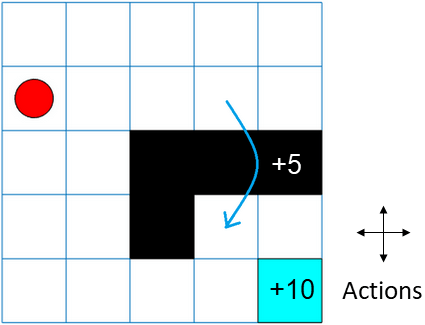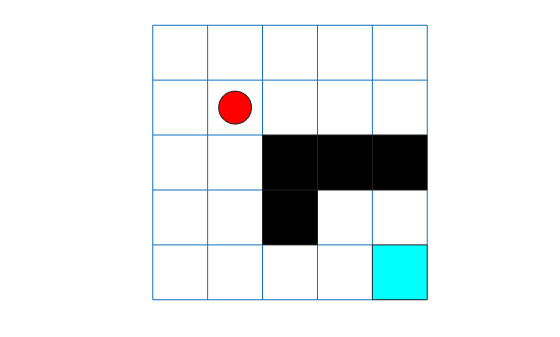# rlMDPEnv

Create Markov decision process environment for reinforcement learning

## Description

A Markov decision process (MDP) is a discrete time stochastic control process. It provides a mathematical framework for modeling decision making in situations where outcomes are partly random and partly under the control of the decision maker. MDPs are useful for studying optimization problems solved using reinforcement learning. Use `rlMDPEnv` to create a Markov decision process environment for reinforcement learning in MATLAB®.

## Creation

### Syntax

``env = rlMDPEnv(MDP)``

### Description

example

````env = rlMDPEnv(MDP)` creates a reinforcement learning environment `env` with the specified `MDP` model.```

### Input Arguments

expand all

Markov decision process model, specified as one of the following:

## Properties

expand all

Markov decision process model, specified as a `GridWorld` object or `GenericMDP` object.

Reset function, specified as a function handle.

## Object Functions

 `getActionInfo` Obtain action data specifications from reinforcement learning environment or agent `getObservationInfo` Obtain observation data specifications from reinforcement learning environment or agent `sim` Simulate trained reinforcement learning agents within specified environment `train` Train reinforcement learning agents within a specified environment `validateEnvironment` Validate custom reinforcement learning environment

## Examples

collapse all

For this example, consider a 5-by-5 grid world with the following rules:

1. A 5-by-5 grid world bounded by borders, with 4 possible actions (North = 1, South = 2, East = 3, West = 4).

2. The agent begins from cell [2,1] (second row, first column).

3. The agent receives reward +10 if it reaches the terminal state at cell [5,5] (blue).

4. The environment contains a special jump from cell [2,4] to cell [4,4] with +5 reward.

5. The agent is blocked by obstacles in cells [3,3], [3,4], [3,5] and [4,3] (black cells).

6. All other actions result in -1 reward.First, create a `GridWorld` object using the `createGridWorld` function.

`GW = createGridWorld(5,5)`
```GW = GridWorld with properties: GridSize: [5 5] CurrentState: "[1,1]" States: [25x1 string] Actions: [4x1 string] T: [25x25x4 double] R: [25x25x4 double] ObstacleStates: [0x1 string] TerminalStates: [0x1 string] ```

Now, set the initial, terminal and obstacle states.

```GW.CurrentState = '[2,1]'; GW.TerminalStates = '[5,5]'; GW.ObstacleStates = ["[3,3]";"[3,4]";"[3,5]";"[4,3]"];```

Update the state transition matrix for the obstacle states and set the jump rule over the obstacle states.

```updateStateTranstionForObstacles(GW) GW.T(state2idx(GW,"[2,4]"),:,:) = 0; GW.T(state2idx(GW,"[2,4]"),state2idx(GW,"[4,4]"),:) = 1;```

Next, define the rewards in the reward transition matrix.

```nS = numel(GW.States); nA = numel(GW.Actions); GW.R = -1*ones(nS,nS,nA); GW.R(state2idx(GW,"[2,4]"),state2idx(GW,"[4,4]"),:) = 5; GW.R(:,state2idx(GW,GW.TerminalStates),:) = 10;```

Now, use `rlMDPEnv` to create a grid world environment using the `GridWorld` object `GW`.

`env = rlMDPEnv(GW)`
```env = rlMDPEnv with properties: Model: [1x1 rl.env.GridWorld] ResetFcn: [] ```

You can visualize the grid world environment using the `plot` function.

`plot(env)`